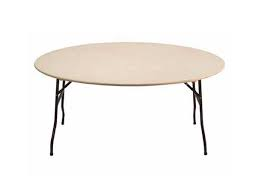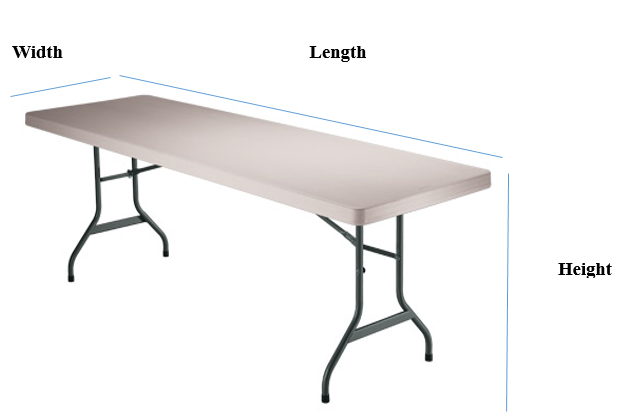### Top tips to having the correct Tablecloth

Knowing how to ask for the correct size tablecloth can be a task if you are not sure what you are looking for.

So look no further, we  have put together a foolproof way,  to measure your table and hire the perfect cloth.

# For a Round Table:

Step 1 : Measure the diameter to the table top.

Step 2: Add 30" to the diameter, this will give you a drop half way to the floor,

OR

for a more formal occasion add 60" to the diameter of the table.Measure the diameter of the Table

Example:

Diameter of table: 60" (5ft round table)

Sum: 60" + 30"= 90"

Tablecloth Required: 90" Round for half way drop.

# Measuring a Square or Rectangle Table:

When measuring your square or rectangle table, the same principles apply.

Step1: Measure the height of the table (this is best measured inches) .

Step2: Measure the length of the Table (measure in inches)

Step 3: Measure the width of the table (measure in inches)

Now you have all the measurements of the table, to work out the perfect tablecloth size, follow this simple sum:

2 x height + length = (this will be the length of the table cloth you will need)
2 x height + width = ( this is the width of the tablecloth, which will cover both ends of the table)## Need Help? .... Give us a call

If you are finding yourself all caught up in the tape measure, then pick up the phone and give our team a call on 01268 691222, we can offer advise and help you select the correct size, shape and colour tablecloth.